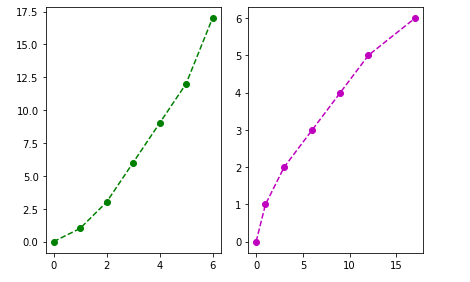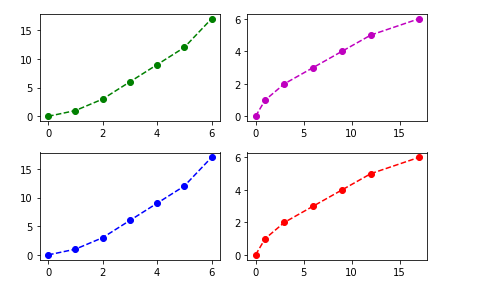# Python | Matplotlib Sub plotting using object oriented API

Plotting using Object Oriented(OO) API in matplotlib is an easy approach to plot graphs and other data visualization methods.

The simple syntax to create the class and object for sub-plotting is –

class_name, object_name = matplotlib.pyplot.subplots(‘no_of_rows’, ‘no_of_columns’)

Let’s take some examples to make it more clear.

Example #1:

 `# importing the matplotlib library ` `import` `matplotlib.pyplot as plt ` ` `  `# defining the values of X ` `x ``=``[``0``, ``1``, ``2``, ``3``, ``4``, ``5``, ``6``] ` ` `  `# defining the value of Y ` `y ``=``[``0``, ``1``, ``3``, ``6``, ``9``, ``12``, ``17``] ` ` `  `# creating the canvas with class 'fig' ` `# and it's object 'axes' with '1' row  ` `# and '2' columns ` `fig, axes ``=` `plt.subplots(``1``, ``2``) ` ` `  `# plotting graph for 1st column ` `axes[``0``].plot(x, y, ``'g--o'``) ` ` `  `# plotting graph for second column ` `axes[``1``].plot(y, x, ``'m--o'``) ` ` `  `# Gives a clean look to the graphs ` `fig.tight_layout() `

Output :In the above example, we used ‘axes'(the object of the class ‘fig’) as an array at the time of plotting graph, it is because when we define the number of rows and columns then array of the objects is created with ‘n’ number of elements where ‘n’ is the product of rows and columns, so if we have 2 columns and two rows then there will be array of 4 elements.

Example #2:

 `# importing the matplotlib library ` `import` `matplotlib.pyplot as plt ` ` `  `# defining the values of X ` `x ``=``[``0``, ``1``, ``2``, ``3``, ``4``, ``5``, ``6``] ` ` `  `# defining the value of Y ` `y ``=``[``0``, ``1``, ``3``, ``6``, ``9``, ``12``, ``17``] ` ` `  `# creating the canvas with class 'fig' ` `# and it's object 'axes' with '1' row  ` `# and '2' columns ` `fig, axes ``=` `plt.subplots(``2``, ``2``) ` ` `  `# plotting graph for 1st element ` `axes[``0``, ``0``].plot(x, y, ``'g--o'``) ` ` `  `# plotting graph for 2nd element ` `axes[``0``, ``1``].plot(y, x, ``'m--o'``) ` ` `  `# plotting graph for 3rd element ` `axes[``1``, ``0``].plot(x, y, ``'b--o'``) ` ` `  `# plotting graph for 4th element ` `axes[``1``, ``1``].plot(y, x, ``'r--o'``) ` ` `  `# Gives a clean look to the graphs ` `fig.tight_layout() `

Output :My Personal Notes arrow_drop_upIf you like GeeksforGeeks and would like to contribute, you can also write an article using contribute.geeksforgeeks.org or mail your article to contribute@geeksforgeeks.org. See your article appearing on the GeeksforGeeks main page and help other Geeks.

Please Improve this article if you find anything incorrect by clicking on the "Improve Article" button below.

Improved By : nidhi_biet

Article Tags :

Be the First to upvote.

Please write to us at contribute@geeksforgeeks.org to report any issue with the above content.